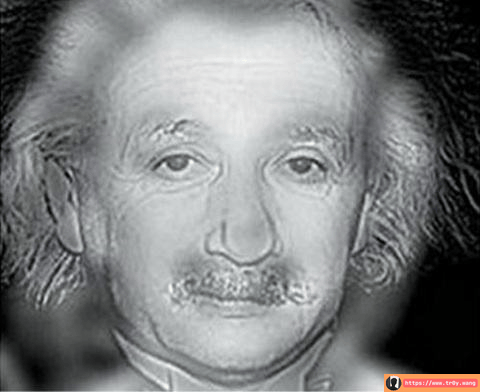Tr0y 6月 12, 2017 23:02:40 本文共 371 字
• 文为知己者书
•• 在其它设备中阅读本文章
•MATLAB 代码

g1=rgb2gray(I1);
s=fftshift(fft2(g1));
[M,N]=size(s);
n1=fix(M/2);
n2=fix(N/2);

% 理想低通滤波器取 d0=10  （15,30）可变
d0=10;
for i=1:M
for j=1:N
d=sqrt((i-n1)^2+(j-n2)^2);
if d<d0
h=1;
else
h=0;
end
s(i,j)=h*s(i,j);
end
end
s=ifftshift(s);
s=uint8(real(ifft2(s)));
figure(1);
imshow(s);

% 爱因斯坦图片处理
g2=rgb2gray(I2);
s2=fftshift(fft2(g2));
[M2,N2]=size(s2);
n12=fix(M2/2);
n22=fix(N2/2);

% 理想高通滤波器取 d02=5  （15,30）可变
d02=5;
for i=1:M2
for j=1:N2
d=sqrt((i-n12)^2+(j-n22)^2);
if d<d02
h=0;
else
h=1;
end
s2(i,j)=h*s2(i,j);
end
end
s2=ifftshift(s2);
s2=uint8(real(ifft2(s2)));
figure(2);
imshow(s2);

% 图片合并
figure(3);
imshow(s3);
imwrite(s3,'he.jpg','JPG')End

What do you think?